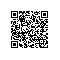# ConcurrentModificationException

package test;
import java.util.Enumeration;
import java.util.Hashtable;
import java.util.Iterator;
import org.junit.Before;
import org.junit.Test;
public class HasttableTest {
private Hashtable<String, String> table;
private int i;
@Before
public void setUp() throws Exception {
i = 0;
table = new Hashtable<String, String>();
table.put("a", "aa");
table.put("b", "bb");
table.put("c", "cc");
}
@Test
public void testEnu() {
System.out.println("Enumeration 遍历开始");
Enumeration<String> keys = table.keys();
while (keys.hasMoreElements()) {
i++;
String key = (String) keys.nextElement();
System.out.println(key + "---" + table.get(key));
System.out.println("i=" + i);
if (i == 1) {
table.put("d", "dd");
table.remove("b");
}
}
System.out.println("Enumeration 遍历结束");
}
@Test
public void TestIte() {
System.out.println("Iterator遍 历开始");
Iterator<String> it = table.keySet().iterator();
while (it.hasNext()) {
i++;
String key = (String) it.next();
System.out.println(key + "---" + table.get(key));
System.out.println("i=" + i);
if (i == 1) {
// table.put("d", "dd");
table.remove("c");
}
}
System.out.println("Iterator 遍历结束");
}
}